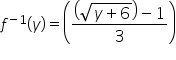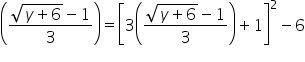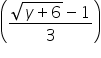# NCRT Solution Exercise 3

Question 1: Let f: {1, 3, 4} → {1, 2, 5} and g: {1, 2, 5} → {1, 3} be given by f = {(1, 2), (3,

5), (4, 1)} and g = {(1, 3), (2, 3), (5, 1)}. Write down gof.

The functions f: {1, 3, 4} → {1, 2, 5} and g: {1, 2, 5} → {1, 3} are defined as

f = {(1, 2), (3, 5), (4, 1)} and g = {(1, 3), (2, 3), (5, 1)}.

gof(1) = g[f(1)] = g(2) = 3 [as f(1) = 2 and g(2) = 3]

gof(3) = g[f(3)] = g(5) = 1 [as f(3) = 5 and g(5) = 1]

gof(4) = g[f(4)] = g(1) = 3 [as f(4) = 1 and g(1) = 3]

∴ gof = {(1, 3), (3, 1), (4, 3)}

Question 2: Let f, g and h be functions from R to R. Show that

(f + g)oh = foh + goh

(f.g)oh = (foh).(goh)

To prove: (f + g)oh = foh + goh

LHS =  [(f + g)oh](x)

= (f + g)[h(x)]

= f [h(x)] + g[h(x)]

= (foh)(x) + (goh)(x)

= {(foh)(x) + (goh)}(x) = RHS

∴ {(f + g)oh}(x) = {(foh)(x) + (goh)}(x) for all x ∈R

Hence, (f + g)oh = foh + goh

To Prove: (f.g)oh = (foh).(goh)

LHS = [(f.g)oh](x)

= (f.g)[h(x)]

= f[h(x)] . g[h(x)]

= (foh)(x) . (goh)(x)

= {(foh).(goh)}(x)  = RHS

∴[(f.g)oh](x) = {(foh).(goh)}(x)      for all x ∈R

Hence, (f.g)oh = (foh).(goh)

Question 3: Find gof and fog, if

(i)  f(x) = |x| and g(x) = |5x − 2|

(ii) f(x) = 8x3 and g(x) = x1/3

(i). f(x) = |x| and g(x) = |5x-2|

∴gof(x) = g(f(x)) = g(|x|) = |5|x|-2|

fog(x) = f(g(x)) = f(|5x-2|) = ||5x-2|| = |5x-2|

(ii). f(x) = 8x3 and g(x) = x1/3

∴gof(x) = g(f(x)) = g(8x3) = (8x3)1/3 = 2x

fog(x) = f(g(x)) = f(x1/3) = 8(x1/3)3 = 8x

Question 4: If f(x) =(4x+3)/(6x−4), x ≠ 2/3, show that fof(x) = x, for all x ≠2/3. What is the inverse of f ?

It is given that f(x) =(4x+3)/(6x-4), x ≠2/3

(fof)(x) = f(f(x)) = f ((4x + 3)/(6x-4))== (16x + 12 + 18x-12) / (24x + 18-24x + 16) = (34x / 34)

= x

∴fof(x) = x, for all x ≠2/3.

⇒ fof = Ix

Hence, the given function f is invertible and the inverse of f is f itself.

Question 5: State with reason whether following functions have inverse

(i) f: {1, 2, 3, 4} → {10} with

f = {(1, 10), (2, 10), (3, 10), (4, 10)}

(ii) g: {5, 6, 7, 8} → {1, 2, 3, 4} with

g = {(5, 4), (6, 3), (7, 4), (8, 2)}

(iii) h: {2, 3, 4, 5} → {7, 9, 11, 13} with

h = {(2, 7), (3, 9), (4, 11), (5, 13)}

(i) f: {1, 2, 3, 4} → {10} defined as f = {(1, 10), (2, 10), (3, 10), (4, 10)}

From the given definition of f, we can see that f is a many one function as

f(1) = f(2) = f(3) = f(4) = 10

∴f is not one – one.

Hence, function f does not have an inverse.

(ii) g: {5, 6, 7, 8} → {1, 2, 3, 4} defined as

g = {(5, 4), (6, 3), (7, 4), (8, 2)}

From the given definition of g, it is seen that g is a many one function as

g(5) = g(7) = 4.

∴ g is not one – one.

Hence, function g does not have an inverse.

(iii) h: {2, 3, 4, 5} → {7, 9, 11, 13} defined as

h = {(2, 7), (3, 9), (4, 11), (5, 13)}

It is seen that all distinct elements of the set {2, 3, 4, 5} have distinct images under h.

∴ Function h is one – one.

Also, h is onto since for every element y of the set {7, 9, 11, 13}, there exists an element x in the set {2, 3, 4, 5}, such that h(x) = y.

Thus, h is a one – one and onto function.

Hence, h has an inverse.

Question 6: Show that f: [−1, 1] → R, given byf(x) = (x / (x+2)) is one – one. Find the inverse of the function f: [−1, 1] → Range f.

(Hint: For y ∈ Range f, y = f(x) =(x/(x+2)), for some x in [−1, 1], i.e., x =(2y/(1−y)

f: [−1, 1] → R is given as f(x) =x(x+2)

For one – one

Let f(x) = f(y)

⇒(x/x+2) = (y/y+2)

⇒xy +2x = xy +2y

⇒2x = 2y

⇒x = y

∴ f is a one – one function.

It is clear that f: [−1, 1] → Range f is onto.

∴ f: [−1, 1] → Range f is one – one and onto and therefore, the inverse of the

function f: [−1, 1] → Range f exists.

Let g: Range f → [−1, 1] be the inverse of f.

Let y be an arbitrary element of range f.

Since f: [−1, 1] → Range f is onto, we have

y = f(x) for some x ∈ [−1, 1]

⇒ y = (x/x+2)

⇒ xy + 2y = x

⇒ x(1-y) = 2y

⇒ x =(2y/1-y), y ≠ 1

Now, let us define g: Range f → [−1, 1] as

g(y) =(2y/1-y), y ≠ 1

Now,

(gof)(x) = g(f(x)) = g (x/(x + 2))=∴ gof = x = I[-1,1] and fog = y = IRange f

∴ f-1 = g

⇒f-1(y) =(2y/(1-y)),         y ≠ 1

Question 7: Consider f: R → R given by f(x) = 4x + 3. Show that f is invertible. Find the

inverse of f.

f: R → R is given by, f(x) = 4x + 3

For one – one

Let f(x) = f(y)

⇒ 4x + 3 = 4y + 3

⇒ 4x = 4y

⇒ x = y

∴ f is a one – one function.

For onto

For y ∈ R, let y = 4x + 3.

⇒ x = ((y-3)/4) ∈ R

Therefore, for any y ∈ R, there exists x = (y-3)/4)) ∈ R, such that

f(x) = f ((y-3)/4)) = 4 ((y-3)/4)) + 3 = y.

∴ f is onto.

Thus,  f is one – one and onto and therefore,  f

−1

exists.

Let us define g: R → R by g(x) =((y-3)/4)

Now,

(gof)(x) = g(f(x)) = g(4x + 3) =((4x + 3)-3) /4 = 4x/4= x

and

(fog)(y) = f(g(y)) = f ((y-3)/4) = 4 ((y-3)/4)) + 3 = y-3 + 3 = y

∴ gof = fog = IR

Hence, f is invertible and the inverse of  f is given by f-1(y) = g(y) =((y-3)/4).

Question 8: Consider f: R+ → [4, ∞) given by f(x) = x2+ 4. Show that f is invertible with the inverse f−1of given f  byf−1(y) = (y – 4)1/2, where ðÂ‘¹+ is the set of all nonnegative real numbers.

f: R+ → [4, ∞) is given as f(x) = x2+ 4.

For one – one

Let f(x) = f(y)

⇒ x2 + 4 = y2 + 4

⇒ x2 = y2

⇒ x = y                [as  x = y ∈ R+]

∴ f is a one – one function.

For onto

For y ∈ [4, ∞), let y = x2+ 4

⇒x2 = y − 4 ≥ 0                              [as y ≥ 4]

⇒ x = (y-4)1/2 ≥ 0

Therefore, for any y ∈ [4, ∞), there exists x = (y-4)1/2 ∈ R+, such that

f(x) = f(y-4)1/2 = ((y-4)1/2 )2+ 4 = y-4 + 4 = y

∴ f is onto.

Thus, f is one – one and onto and therefore, f

−1

exists.

Let us define g: [4, ∞) → R+ by g(y) = Thus, f is one – one and onto and therefore, f−1exists.

Let us define g: [4, ∞) → R+ by g(y) = (y-4)1/2

Now,

(gof)(x) = g(f(x)) = g(x2 + 4) = ((x2 + 4)-4))1/2 = (x2)1/2 = x

and

(fog)(y) = f(g(y)) = f(y-4 )1/2 = ((y-4 )1/2)2+ 4 = y-4 + 4 = y

∴ gof = fog = IR

Hence, f is invertible and the inverse of f is given by f-1(y) = g(y) = (y-4 )1/2

Question 9: Consider f: R+ → [−5, ∞) given by f(x) = 9x2+ 6x − 5. Show that f is invertible withf: R+ → [−5, ∞) is given as f(x) = 9x2+ 6x − 5.

Let y be an arbitrary element of [−5, ∞).

Let y = 9x2+ 6x – 5

⇒ y = (3x + 1)2-1-5 = (3x + 1)2-6

⇒ y + 6 = (3x + 1)2

⇒ 3x + 1 = (y + 6)1/2        [as y ≥ −5 ⇒ y + 6 > 0]

⇒ x =((y+6 )1/2-1) / 3)

∴ f is onto, thereby range f = [−5, ∞).

Let us define g: [−5, ∞) → R+ as g(y) =((y+6 )1/2-1) / 3)

Now,

(gof)(x) = g(f(x)) = g(9x2 + 6x-5) = g((3x + 1)2-6)

= ((3x + 1)2-6 + 6)1/2 -1

= (3x + 1-1) /3 = (3x / 3) = x

and

(fog)(y) = f(g(y)) = f= ((y + 6)1/2)2 -6 = y + 6-6 = y

∴ gof = x = IR and fog = y = IRange f

Hence,  f is invertible and the inverse of f is given by

f−1(y) = g(y) =Question 10:

Let f: X → Y be an invertible function. Show that f  has unique inverse. (Hint: suppose g1 and g2 are two inverses of f. Then for all y ∈ Y, fog1(y) = Iy(y) = fog2(y). Use one – one ness of f).

Let f: X → Y be an invertible function.

Also, suppose f has two inverses (sayg1 and g2)

Then, for all y ∈Y, we have

fog1(y) = IY(y) = fog2(y)

⇒ f(g1(y)) = f(g2(y))

⇒ g1(y) = g2(y)                  [as f  is invertible ⇒ f  is one – one]

⇒ g1 = g2                           [as g is one – one]

Hence, f has a unique inverse.

Question 11: Consider f: {1, 2, 3} → {a, b, c} given by f(1) = a, f(2) = b and f(3) = c. Find f−1and show that (f−1)−1= f.

Function f: {1, 2, 3} → {a, b, c} is given by f(1) = a, f(2) = b, and f(3) = c

If we define g: {a, b, c} → {1, 2, 3} as g(a) = 1, g(b) = 2,  g(c) = 3.

We have

(fog)(a) = f(g(a)) = f(1) = a

(fog)(b) = f(g(b)) = f(2) = b

(fog)(c) = f(g(c)) = f(3) = c

and

(gof)(1) = g(f(1)) = f(a) = 1

(gof)(2) = g(f(2)) = f(b) = 2

(gof)(3) = g(f(3)) = f(c) = 3

∴ gof = IX and fog = IY, where X = {1, 2, 3} and Y= {a, b, c}.

Thus, the inverse of f exists and f−1= g.

∴ f−1:{a, b, c} → {1, 2, 3} is given by f−1(a) = 1, f−1(b) = 2, f–1   (c) = 3

Let us now find the inverse of f−1 i.e., find the inverse of g.

If we define h: {1, 2, 3} → {a, b, c} as h(1) = a, h(2) = b, h(3) = c

We have

(goh)(1) = g(h(1)) = g(a) = 1

(goh)(2) = g(h(2)) = g(b) = 2

(goh)(3) = g(h(3)) = g(c) = 3

and

(hog)(a) = h(g(a)) = h(1) = a

(hog)(b) = h(g(b)) = h(2) = b

(hog)(c) = h(g(c)) = h(3) = c

∴ goh = IX and hog = IY, where X = {1, 2, 3} and Y = {a, b, c}.

Thus, the inverse of g exists and g−1= h ⇒ (f−1)−1= h.

It can be noted that h = f.

Hence, (f−1)−1= f.

Question 12:

Let f: X → Y be an invertible function. Show that the inverse of f−1 is f,

i.e., (f−1)−1= f.

Let f: X → Y be an invertible function.

Then, there exists a function g: Y → X such that gof = IX and fog = IY

Here, f−1= g.

Now, gof = IX and fog = IY

⇒ f−1of = IX and fof−1= IY

Hence, f−1: Y → X is invertible and f is the inverse of f−1 i.e., (f−1)−1= f.

Question 13: If f: R → R be given by f(x) = (3 − x3)1/3, then fof(x) is

(A) (1/x3)           (B) x3                   (C) x                     (D) (3 − x3)

f: R → R be given as f(x) = (3-x3)1/3

∴ fof(x) = f(f(x)) = f= [3-(3-x3)]1/3 = (x3)1/3 = x

∴ fof(x) = x

Question 14: Let f: R- {-4/3} → R be a function as f(x) =(4x / (3x+4)). The inverse of f is map g: Range f → R- {-4/3} given by

(A) g(y) =(3y / (3-4y))

(B) g(y) =(4y / (4-3y))

(C) g(y) =(4y / (3-4y))

(D) g(y) =(3y / (4-3y))

It is given that f: R − {−4/3} → R be a function as f(x) = (4x / (3x+4))

Let y be an arbitrary element of Range f.

Then, there exists x ∈ R- {-4/3} such that y = f(x)

⇒ y = (4x / (3x + 4))

⇒ 3xy + 4y = 4x

⇒ x(4-3y) = 4y

⇒ x = (4y / (4-3y))

Let us define g: Range f → R- {-4/3} as g(y) = (4y / (4-3y))

Now,

Now,

gof (x)= g(f(x)) ==(16x / (12x + 16 – 12x)) =(16x / 16) = x

and

fog (y)= f(g(y)) ==(16y / (12y + 16 – 12y)) =(16y / 16) = y

∴ gof = IR-{-4/3}and fog = IRange f

Thus, g is the inverse of f i.e., f−1= g.

Hence, the inverse of f is the map g: Range f → R- {-4/3}, which is given by g(y) = (4y / (4-3y)).